# Equation Solver

100K+Everyone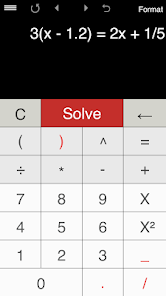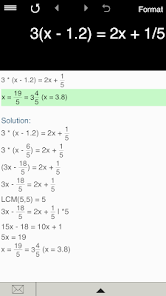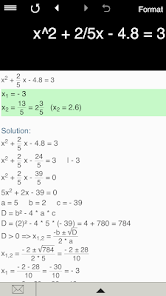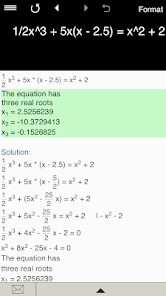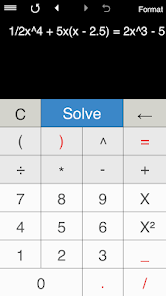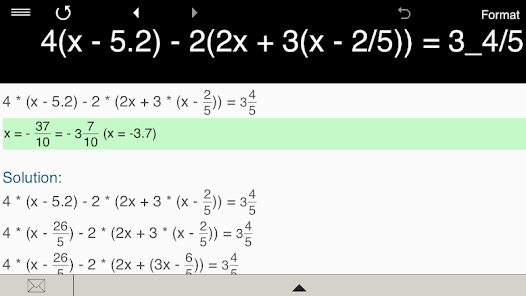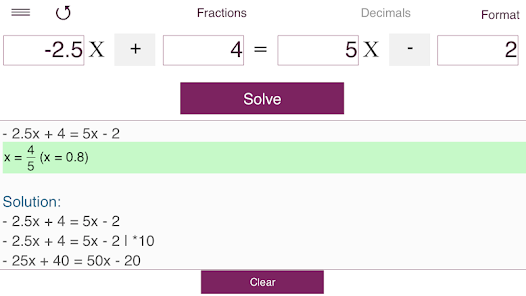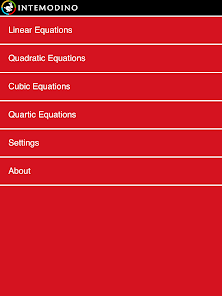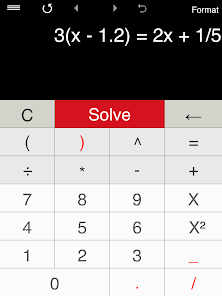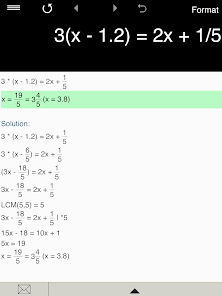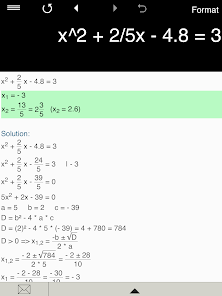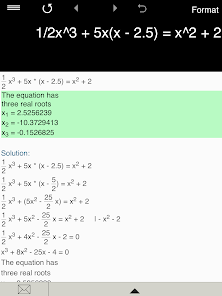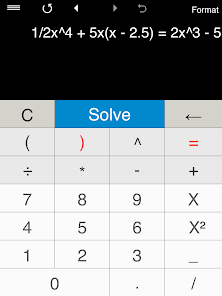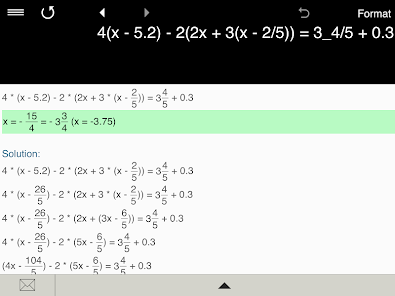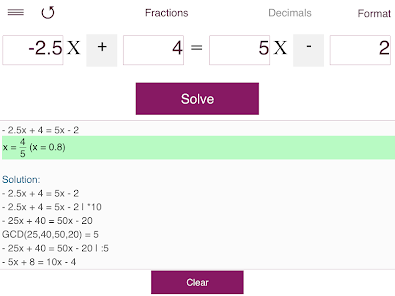Equation Calculator 4-in-1 is the ultimate app that helps you solve linear equations in one variable, quadratic equations with real and complex roots, cubic and quartic equations. It's intuitive and easy to use. Just type in any equation you want to solve and Equation Calculator will show you the result.

Everything you need to solve equations or check results!

Features:

• Linear Equation Solver.
• Quadratic Equation Solver.
• Cubic Equation Solver.
• Quartic Equation Solver.
• Solves equations with fractions and parentheses. Note: the app doesn’t yet support equations with a variable in the denominator.
• Supports integers, fractions and decimals, including negative values.
• Predefined formats.
• Detailed step-by-step instructions for linear equations and quadratic equations.
• Stores history with the possibility to recall previous equations.
• Back and forward buttons to check and recall recent equations.
• Sends results and history via email.
Updated on
Jul 29, 2020

## Data safety

Developers can show information here about how their app collects and uses your data. Learn more about data safetyNo information available